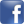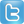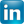Math in the Best of the Web Directory

# Math

#### Top/Kids and Teens/Science and Math/Math

• 10on10 Math for Kids

Introduces a math learning program for 4-12 year old kids featuring the use of creative worksheets categorized according to a child's ability and age.

• A Maths Dictionary for Kids

Interactive dictionary of mathematical terms for children, with colorful explanation and opportunities to try things out.

• A+ Math

Features a game room, flashcards, and a math word find puzzle. Dedicated to interactively making kids improve on their math skills.

• abcteach - Math

Learn basic math skills, including addition, subtraction, multiplication, and division, as well as telling time and handling money.

• All Elementary Mathematics

Serves as an online mathematical high school. Presents math theories, problems, tests, references, and learning helps.

Dr. Math is available to answer math question for students in elementary school and all the way up to college and beyond.

• Awesome Library - Math

Learn math for the appropriate grade level, as well as pre-algebra, algebra, data analysis, and statistics. Check out the games, too.

• Basic-Mathematics.com

Provides math lessons and interactive calculators.

• Binary Math

A complete resource for binary math. Learn about binary numbers, its arithmetic, and conversions. Practice problems are also available.

• Convertalot

Provides converters and online calculators, most of which are interactive.

• Coolmath 4 Kids

Interactive page containing math tools specially designed for kids. Includes math games, puzzles, and lessons.

• Crickweb

Educational interactive math resource for primary school children, plus fun games for ages 4 to 11.

• Elementary Math Games

Learn with free math games, worksheets, and flash cards.

• EnchantedLearning.com - Math Dictionary

Glossary defines lots of math-related terms, from "abacus" to "zigzag," with diagrams and illustrations.

• Free Math Help

Provides lessons and interactive problems in algebra, geometry, trigonometry, and calculus. For grades 1 through 11.

• I Know It

Features online math games for kids in first through fifth grades. Topics include fractions, geometry, telling time, addition, multiplication, counting money, and place value.

• iCoachMath.com

Features a math dictionary and resources for elementary, middle school, and high school math.

• IXL Learning

Math program for grades K-8 that assesses students' understanding as they practice, and generates detailed performance reports.

• Johnnie's Math Page

Features a wide variety of math activities and resources for kids and their teachers. With worksheets, puzzles and games.

• Kids Math Games

Offers an interactive and interesting way of learning math. Features worksheets, videos, quizzes, pictures, and activities.

• KidsNumbers.com

Check out the Magic Math Box, Spatial Math, flash cards, calculator, and Math Foundations.

• Math Apprentice

Offers interactive jobs that use different math concepts. Includes percentages, geometry, money, time, and measurement.

• Math Cats

Features a variety of games, fun facts and tools to help make math fun for younger children.

• The Math Forum: Student Center

Directory of math information sorted by grade level.

• Math Glossary

Multimedia presentation math terms with sorted presented according to grade level.

• Math Goodies

Math help site featuring interactive lessons, puzzles and worksheets.

• Math Homework Help

Free math homework resource site for students in Grades 7 to 10. Features interactive tutorials and an online scientific calculator.• Math is Fun

Offers mathematics as well as some fun games and puzzles to increase basic math skills.

• Math Online

Provides games, practice, tests, tips, problems, puzzles, and learning tools for elementary, middle, and high school learners.

• Math Reference Tables

Math tables, covers algebra formulas, geometry, trigonometry, discrete/linear, statistics, calculus, and advanced subjects.

• Math Trail

• Math.com

Provides math lessons and math homework help from basic math to algebra.

• Math-Aids.com

Free math resource for parents, teachers, students, and homeschoolers. Offers worksheets for various math topics.

• Math-a-rama

Grade level category sorted resource providing math tools, references, and resources.

• Mathematics and Games

Math training program for children of all ages featuring learning activities in elementary arithmetic, logic games, and exercises.

• MathNook

Offers free online math games that target a variety of skills.

• NCES Kids Zone

Government site provides information and resources designed to help kids find a college or library.

• Percentage Calculator

Simple online tool for calculating calculating the percentage of a particular number.

• Reflex Math Fact Fluency

A game-based system that helps students of all ability levels to develop instant recall of basic math facts. Offers a free trial.

• Splash Math

Award winning math program for grade school age children. Site offers online games, information on the program, and a free online trial.• Thinking Blocks

Program for kids in grades 1 to 5 to use for learning to model and solve math problems.

• Vancouver Math Tutor

Contains a variety of math resources for students of all ages, parents, and aspiring tutors.

• WebMath

Explores topics in arithmetic, K-8 math, trigonometry, calculus, and everything in between.

• Who Wants Pizza?

Using slices of pizza, Cynthia Lanius offers elementary age kids a way to connect and learn about fractions.

• Yummy Math

Relevant real life mathematics problems for kids to solve.